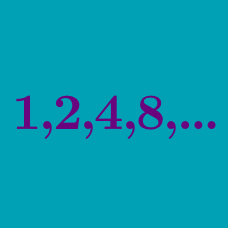Algebra

# Geometric Progression Sum

Evaluate

$1 + 2 + 2^2 + 2^3 + \ldots + 2^{ 24 }.$

A bookshelf has $$6$$ shelves. The top shelf has $$2$$ books, and each other shelf has $$3$$ times as many books as the shelf directly above it. If you want to read a book from the shelves, how many choices do you have?

Details and assumptions

All of the books are different.

The series $$a_1, a_2, a_3, a_4, a_5, a_6, a_7$$ is a geometric progression with $$a_4 = 48$$ and $$a_5 = 96$$. What is the sum of the these $$7$$ terms?

Details and assumptions

A geometric progression is a sequence of numbers, in which each subsequent number is obtained by multiplying the previous number by a common ratio / multiple. For example, $$1, 2, 4, 8, 16, 32, 64, \ldots$$ is a geometric progression with initial term 1 and common ratio 2.

Consider a geometric progression with initial term $$4$$ and common ratio $$5$$. What is the smallest value of $$n$$ for which the sum of the first $$n$$ terms is greater than $$800$$?

Evaluate the sum to infinity:

$\frac{ 14 } { 2^0} + \frac{ 14 } { 2^1 } + \frac{ 14 } { 2^2 } + \ldots .$

×